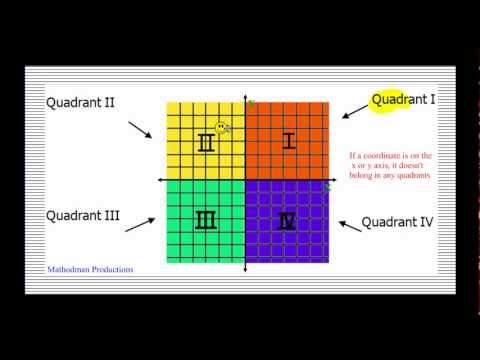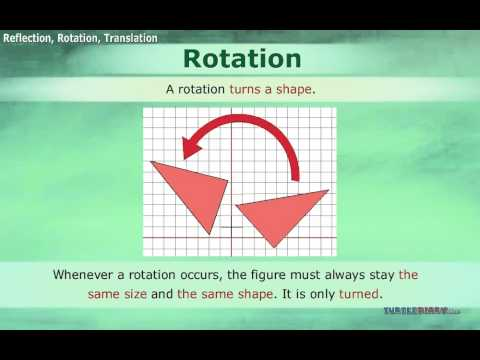## Co-ordinates

Subject: Compulsory Maths

#### Overview

The ordered pair of the number which is used to indicate the position of a point on a grid is known as the coordinates of a point. Co-ordinates axes are the horizontal and vertical number lines which intersect each other perpendicularly at the point O.

#### Co-ordinates

The ordered pair of the number which is used to indicate the position of a point on a grid is known as the coordinates of a point.For example,

The position of the point P on the grid is 5 units along 3 units up is written as (5,3).

Co-ordinates axes are the horizontal and vertical number lines which intersect each other perpendicularly at the point O.

Here, XOX' is called x-axis or abscissa

YOY' is called the y-axis or ordinate.

The point O is called the origin.

Similarly, the interested x-axis and y-axis divide the co-ordinate plane into 4 regions which are known as quadrants.Finding points in all four quadrants

The following are the points which are used to find the points in all four quadrants,

1. In the first quadrant, the co-ordinates of a point are (x,y).
2. In the second quadrant, the co-ordinates of a point are (-x,y)
3. In the third quadrant, the co-ordinates of point are (-x,-y).
4. In the fourth quadrant, the co-ordinates of point (x,-y).
5. The co-ordinates of the origin are (0,0).
6. The co-ordinates of any point on the x-axis are (x,0) or (-x,0).
7. The co-ordinates of any point on the y-axis are (0,y) or (0,-y).

Plotting points in all four quadrants

##### Things to remember
• The following are the points which are used to find the points in all four quadrants,

1. In the first quadrant, the co-ordinates of a point are (x,y).
2. In the second quadrant, the co-ordinates of a point are (-x,y)
3. In the third quadrant, the co-ordinates of point are (-x,-y).
4. In the fourth quadrant, the co-ordinates of point (x,-y).
5. The co-ordinates of the origin are (0,0).
6. The co-ordinates of any point on the x-axis are (x,0) or (-x,0).
7. The co-ordinates of any point on the y-axis are (0,y) or (0,-y).
• It includes every relationship which established among the people.
• There can be more than one community in a society. Community smaller than society.
• It is a network of social relationships which cannot see or touched.
• common interests and common objectives are not necessary for society.
##### Videos for Co-ordinates##### Coordinate Plane and Plotting Points##### Translations Reflections and Rotations

The system of describing a position of a point by using coordinates was invented by  French Mathematician called Rene Descartes in the 17th century.

The coordinate axes are mentioned below:

• XOX' is called the x-axis or abscissa.
• YOY' is called the y-axis or ordinate.
• The point O is called the origin.

Similarly, The quadrants of coordinate are mentioned below:

• The region XOY is called the first quadrant.
• The region X'OY is called the second quadrant.
• The region X'OY' is called the third quadrant.
• The region XOY' is called the fourth quadrant.

Solution:

Given point, A(2,3)
Since both x and y coordinates are positive, so it lies in the first quadrant.

Also, Given points, B(-3,2)}
Since x-coordinate is negative and y-coordinate is positive, so it lies in the second quadrant.

Similarly, Given points, C(-2,-3)
Since both x and y coordinates are negative, so it lies in the third quadrant.

And, Given points, D(3,-2)
Since x-coordinate is positive and y-coordinate is negative, so it lies in the fourth quadrant.